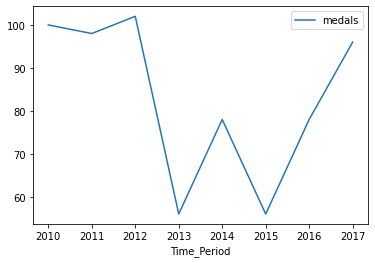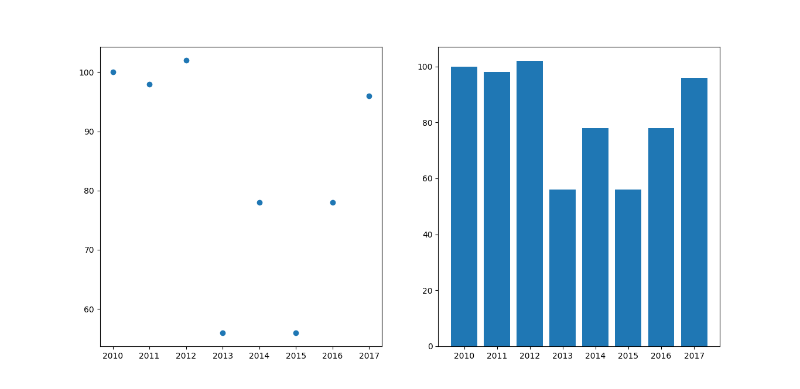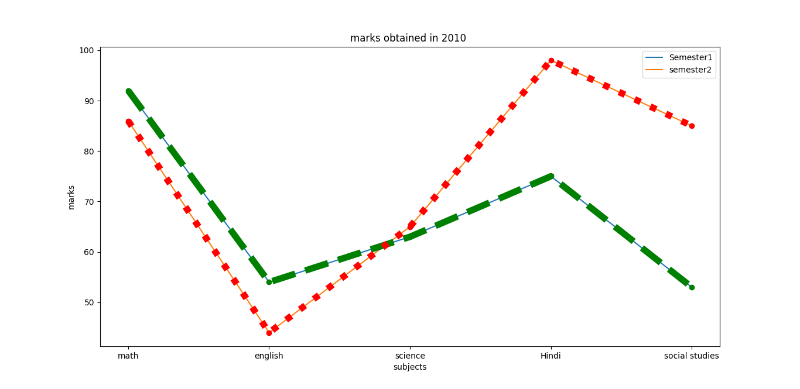# Plotting a trend graph in Python

• Last Updated : 21 Apr, 2021

Prerequisites: Matplotlib

A trend Graph is a graph that is used to show the trends data over a period of time. It describes a functional representation of two variables (x , y). In which the x is the time-dependent variable whereas y is the collected data.  The graph can be in shown any form that can be via line chart, Histograms, scatter plot, bar chart, and pie-chart. In python, we can plot these trend graphs by using matplotlib.pyplot library. It is used for plotting a figure for the given data.

Attention geek! Strengthen your foundations with the Python Programming Foundation Course and learn the basics.

To begin with, your interview preparations Enhance your Data Structures concepts with the Python DS Course. And to begin with your Machine Learning Journey, join the Machine Learning - Basic Level Course

The task is simple and straightforward, for plotting any graph we must suffice the basic data requirement after this determine the values of x over the period of time and data collected for y. Plot the graphs for the above-given data.

Given below are various implementations to depict the same:

Example 1:

## Python3

 `# import all the libraries``import` `numpy as np``import` `pandas as pd``import` `matplotlib.pyplot as plt`` ` `# create a dataframe``Sports ``=` `{``    ``"medals"``: [``100``, ``98``, ``102``, ``56``, ``78``, ``56``, ``78``, ``96``],``    ``"Time_Period"``: [``2010``, ``2011``, ``2012``, ``2013``, ``2014``, ``2015``, ``2016``, ``2017``]``}`` ` `df ``=` `pd.DataFrame(Sports)``print``(df)`` ` `# to plot the graph``df.plot(x``=``"Time_Period"``, y``=``"medals"``, kind``=``"line"``)``plt.show()`

Output:

```   medals  Time_Period
0     100         2010
1      98         2011
2     102         2012
3      56         2013
4      78         2014
5      56         2015
6      78         2016
7      96         2017```Example 2: Using the above data we would plot the scatter and bar graph.

## Python3

 `# import all the libraries``import` `numpy as np``import` `pandas as pd``import` `matplotlib.pyplot as plt`` ` `# create a dataframe``Sports ``=` `{``    ``"medals"``: [``100``, ``98``, ``102``, ``56``, ``78``, ``56``,``               ``78``, ``96``],``   ` `    ``"Time_Period"``: [``2010``, ``2011``, ``2012``, ``2013``,``                    ``2014``, ``2015``, ``2016``, ``2017``]``}``df ``=` `pd.DataFrame(Sports)``print``(df)`` ` ` ` `# to plot the graph``# subplot (rowno,columno,position) is used``# to plot in a single frame.``# to plot the scatter graph ,write kind= scatter``df.plot(x``=``"Time_Period"``, y``=``"medals"``, kind``=``"scatter"``)``plt.title(``"scatter chart"``)``plt.subplot(``1``, ``1``, ``1``)`` ` ` ` `# to Plot the graph in Bar chart``df.plot(x``=``"Time_Period"``, y``=``"medals"``, kind``=``"bar"``)``plt.title(``"bar"``)``plt.subplot(``1``, ``1``, ``2``)`` ` `plt.show()`

Output:Example 3: student getting marks in 2010.

## Python3

 `# import the library``import` `matplotlib.pyplot as plt`` ` ` ` `# Creation of Data``x1 ``=` `[``'math'``, ``'english'``, ``'science'``, ``'Hindi'``, ``'social studies'``]``y1 ``=` `[``92``, ``54``, ``63``, ``75``, ``53``]``y2 ``=` `[``86``, ``44``, ``65``, ``98``, ``85``]`` ` `# Plotting the Data``plt.plot(x1, y1, label``=``'Semester1'``)``plt.plot(x1, y2, label``=``'semester2'``)`` ` `plt.xlabel(``'subjects'``)``plt.ylabel(``'marks'``)``plt.title(``"marks obtained in 2010"``)`` ` `plt.plot(y1, ``'o:g'``, linestyle``=``'--'``, linewidth``=``'8'``)``plt.plot(y2, ``'o:g'``, linestyle``=``':'``, linewidth``=``'8'``)`` ` `plt.legend()`

Output:My Personal Notes arrow_drop_up